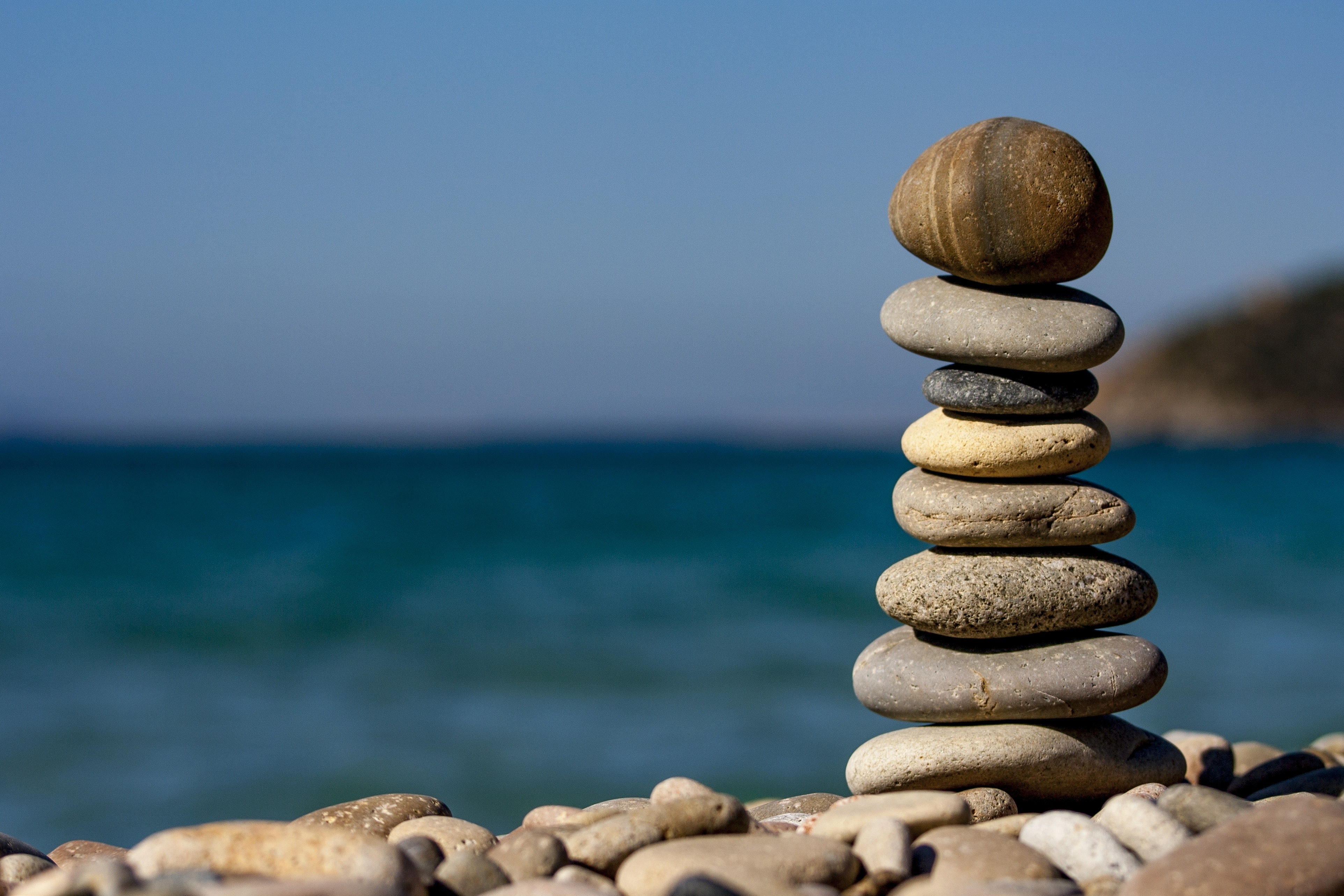# Solution to problems – class 9 – Set 1 Q31, Q32, Q33

Last updated on May 10th, 2021 at 03:49 pm

## Problem Statement

31) If a box is pushed horizontally on the floor with a force of 10 N and it moves 5 meter along the line of action of the force, then what is the work done by the Gravity or earth’s gravitational pull on that box ?

Solution:
The angle between gravity and the displacement is 90 degrees.

So angle theta between force and displacement = 90 degrees here.

As we know, Work done = FS Cos Theta = FS cos 90 = 0
(as cos 90 = 0)
So in this case, work done by gravity is zero.

[Physicsteacher notes: Don’t get confused by the 10 N force mentioned in the problem. Certainly it does ‘Work’ here, being in the same line with the displacement. But the question didn’t ask about that. so we don’t need to consider it in this case.

## Problem Statement

32) A train is moving at a velocity of 25 ms-1. It is brought to rest by applying the brakes which produces a uniform retardation of 0.5 ms-2.
Calculate
(i) the velocity of the train after 10 s
(ii) If the mass of the train is 20000 kg then calculate the force required to stop the train

Solution:
(i) v = u – at = 25 – 0.5X10 = 20 m/s

(ii) mass m = 20000 kg.
we know Force = mass x acceleration = 20000 x 0.5 = 10000 N

## Problem Statement

33) A spring balance is used to find the weight of a body X on the surface of the moon. The mass of the body X is 2 kg and its weight is recorded as 3.4 N. The weight of another body Y recorded by the same balance is found to be 7.65 N. Calculate the mass of the body Y.

See also  Solution to problems - class 9 physics - Set 1 Q 54

Solution:

Body X data on the moon

mass = m=2 kg

Weight = W= 3.4 N

We know weight on the moon can be expressed as, W = m gmoon …….(1)
Here, gmoon = W/m = 3.4/2 = 1.7 m/s2

Body Y data on the moon

Weight = W= 7.65 N

Again from eqn (1), mass of Y= W/gmoon = 7.65/1.7 = 4.5 kg

[Physicsteacher notes: a spring balance may have 2 scales, one showing mass and the other one showing the weight in Newton. However, all spring balances won’t have both.

As Wikipedia states: “A spring balance may be labeled in both units of force (poundals, Newtons) and mass (pounds, kilograms/grams). “ Link: https://en.wikipedia.org/wiki/Spring_scale]

Question Set – Physics Problems for grade 9

Scroll to top
error: physicsTeacher.in